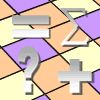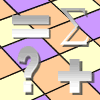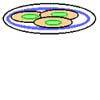Filter by: Content type:
Age range:
Challenge level:

### There are 45 results### Factor-multiple Chains

##### Age 7 to 11Challenge Level

Can you see how these factor-multiple chains work? Find the chain which contains the smallest possible numbers. How about the largest possible numbers?### Substitution Cipher

##### Age 11 to 14Challenge Level

Find the frequency distribution for ordinary English, and use it to help you crack the code.### Excel Technique: Inserting an Increment Button

##### Age 11 to 16Challenge Level

Learn how to use increment buttons and scroll bars to create interactive Excel resources.### Excel Technique: Triangular Arrays by Turning Off Zeros

##### Age 11 to 16Challenge Level

Learn how to use Excel to create triangular arrays.### Excel Technique: Using Paste Special to Lift a Copy of Values

##### Age 11 to 16Challenge Level### Excel Technique: Logic Tests

##### Age 11 to 16Challenge Level

Learn how to use logic tests to create interactive resources using Excel.### Excel Investigation: Target Decimal

##### Age 11 to 16Challenge Level

Use an Excel spreadsheet to approximate a decimal using trial and improvement.### Excel Technique: LOOKUP Functions

##### Age 11 to 16Challenge Level

Learn how to use lookup functions to create exciting interactive Excel spreadsheets.### Excel Investigation: Happy Numbers

##### Age 11 to 16Challenge Level

Take any whole number between 1 and 999, add the squares of the digits to get a new number. Use a spreadsheet to investigate this sequence.### Excel Investigation: Number Pyramids

##### Age 11 to 16Challenge Level

Use Excel to create some number pyramids. How are the numbers in the base line related to each other? Investigate using the spreadsheet.### Excel Investigation: Pascal Multiples

##### Age 11 to 16Challenge Level

This spreadsheet highlights multiples of numbers up to 20 in Pascal's triangle. What patterns can you see?##### Age 11 to 16Challenge Level

Use Excel to investigate remainders and divisors. Was your result predictable?### Excel Technique: Conditional Formatting

##### Age 11 to 16Challenge Level

Learn how to use conditional formatting to create attractive interactive spreadsheets in Excel.### Excel Technique: Composite Bar Charts

##### Age 11 to 16Challenge Level

Learn how to use composite bar charts in Excel.### Excel Investigation: Ring on a String

##### Age 11 to 16Challenge Level

This investigation uses Excel to optimise a characteristic of interest.### Plates of Biscuits

##### Age 7 to 11Challenge Level

Can you rearrange the biscuits on the plates so that the three biscuits on each plate are all different and there is no plate with two biscuits the same as two biscuits on another plate?### Excel Technique: LCM & HCF (or GCD)

##### Age 11 to 16Challenge Level

Learn how to use the Excel functions LCM and GCD.### Excel Investigation: Fraction Combinations

##### Age 11 to 16Challenge Level

Choose four numbers and make two fractions. Use an Excel spreadsheet to investigate their properties. Can you generalise?### Excel Investigation: Multiples of Three

##### Age 11 to 16Challenge Level

Use Excel to investigate digit sums of multiples of three. Can you explain your findings?### Excel Investigation: Planks

##### Age 11 to 16Challenge Level

I have an unlimited supply of planks, of lengths 7 and 9 units. Putting planks end to end, what total lengths can be achieved? Use Excel to investigate.##### Age 11 to 16Challenge Level

A heap of beads was shared out by a professional bead sharer. Use the information given to find out how many beads there were at the start.### Excel Technique: Making a Table for a Function of Two Independent

##### Age 11 to 16Challenge Level

Learn how to make a simple table using Excel.### Excel Interactive Resource: Make a Copy

##### Age 11 to 16Challenge Level

Investigate factors and multiples using this interactive Excel spreadsheet. Use the increment buttons for experimentation and feedback.### Multiples Grid

##### Age 7 to 11Challenge Level

What do the numbers shaded in blue on this hundred square have in common? What do you notice about the pink numbers? How about the shaded numbers in the other squares?### Excel Investigation: Pythagorean Triples

##### Age 11 to 16Challenge Level

Use Excel to find sets of three numbers so that the sum of the squares of the first two is equal to the square of the third.### Excel Investigation: Difference Tuples

##### Age 11 to 16Challenge Level

Use an Excel spreadsheet to investigate differences between four numbers. Which set of start numbers give the longest run before becoming 0 0 0 0?### What's the Weather Like?

##### Age 11 to 14Challenge Level

With access to weather station data, what interesting questions can you investigate?### Excel Investigation: Power Crazy

##### Age 11 to 16Challenge Level

When is 7^n + 3^n a multiple of 10? Use Excel to investigate, and try to explain what you find out.### Excel Investigation: the Difference of Two (same) Powers

##### Age 11 to 16Challenge Level

If you take two integers and look at the difference between the square of each value, there is a nice relationship between the original numbers and that difference. Can you find the pattern using. . . .### Excel Investigation: Sums of Consecutive Numbers

##### Age 11 to 16Challenge Level### Getting to Know Excel

##### Age 11 to 16Challenge Level

A number of useful techniques that can extend your use of Excel.### Excel Interactive Resource: Long Multiplication

##### Age 11 to 16Challenge Level

Use an Excel spreadsheet to explore long multiplication.### Time of Birth

##### Age 11 to 14Challenge Level

A woman was born in a year that was a square number, lived a square number of years and died in a year that was also a square number. When was she born?### Sending a Parcel

##### Age 11 to 14Challenge Level

What is the greatest volume you can get for a rectangular (cuboid) parcel if the maximum combined length and girth are 2 metres?### Excel Interactive Resource: Fraction Addition & Fraction Subtraction

##### Age 11 to 16Challenge Level

Use Excel to practise adding and subtracting fractions.### Excel Interactive Resource: Fraction Multiplication

##### Age 11 to 16Challenge Level

Use Excel to explore multiplication of fractions.### Excel Interactive Resource: Interactive Division

##### Age 11 to 16Challenge Level

Use an Excel to investigate division. Explore the relationships between the process elements using an interactive spreadsheet.### Take Ten Sticks

##### Age 11 to 16Challenge Level

Take ten sticks in heaps any way you like. Make a new heap using one from each of the heaps. By repeating that process could the arrangement 7 - 1 - 1 - 1 ever turn up, except by starting with it?### Excel Interactive Resource: Equivalent Fraction Bars

##### Age 11 to 16Challenge Level

A simple file for the Interactive whiteboard or PC screen, demonstrating equivalent fractions.### Litov's Mean Value Theorem

##### Age 11 to 14Challenge Level

Start with two numbers and generate a sequence where the next number is the mean of the last two numbers...### Excel Interactive Resource: Haitch

##### Age 11 to 16Challenge Level

An Excel spreadsheet with an investigation.### Excel Interactive Resource: Number Grid Functions

##### Age 11 to 16Challenge Level

Use Excel to investigate the effect of translations around a number grid.### Excel Interactive Resource: Multiples Chain

##### Age 11 to 16Challenge Level

Use an interactive Excel spreadsheet to investigate factors and multiples.### Excel Interactive Resource: the up and Down Game

##### Age 11 to 16Challenge Level

Use an interactive Excel spreadsheet to explore number in this exciting game!### Cola Can

##### Age 11 to 14Challenge Level

An aluminium can contains 330 ml of cola. If the can's diameter is 6 cm what is the can's height?